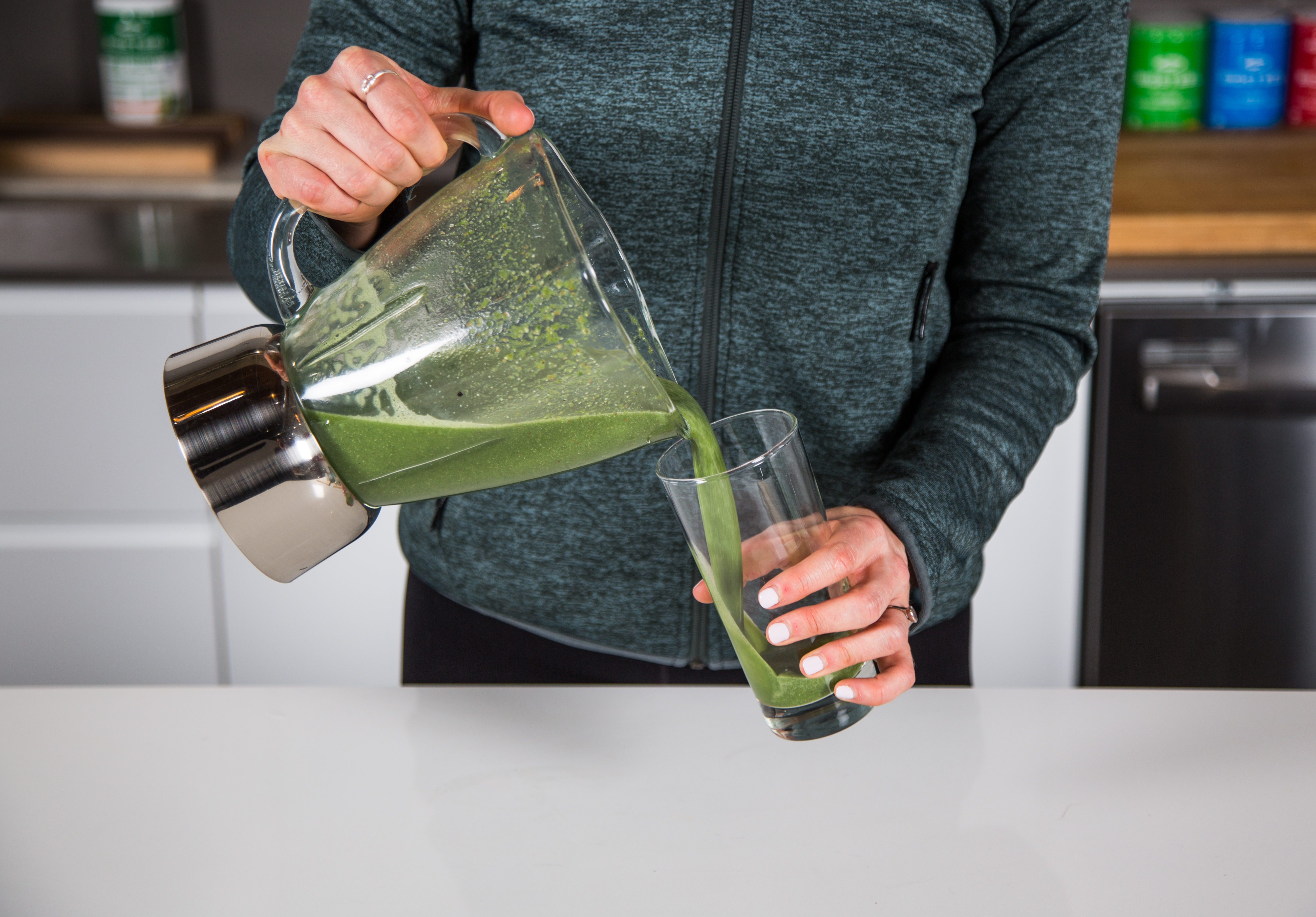# Eugenie Bouchard

##### 07.08.18

Here is a caption.

Here is a caption.

Here is a caption.

Here is a caption.

Here is a caption. Here is a caption. Here is a caption. Here is a caption. Here is a caption. Here is a caption. Here is a caption. Here is a caption. Here is a caption. Here is a caption. Here is a caption. Here is a caption. Here is a caption. Here is a caption. Here is a caption. Here is a caption. Here is a caption. Here is a caption. Here is a caption. Here is a caption. Here is a caption. Here is a caption. Here is a caption. Here is a caption. Here is a caption.

CAPTION THIS.